## Introduction

Linkage disequilibrium, the non-independence of alleles at different loci, has been widely used to infer important historical population events or detect the effect of selection pressure (Barton, 2011 and references therein). Two loci on a chromosome, with alleles A, a and B, b respectively, are said to be in linkage equilibrium, or gametic phase equilibrium, when each haplotype frequency is equal to the product of the corresponding allelic frequencies. Linkage disequilibrium refers to the deviation from this equilibrium and, when linkage phase is known, is measured as a function of the statistics D=pABpApB where pAB is the frequency of the haplotype AB and pA (pB) is the frequency of the A (B) allele.

Various measures have been published in the literature for assessing statistical association between alleles at different loci (see for instance Hedrick, 1987 for a comparative study of six measures for diallelic loci and Cierco-Ayrolles et al., 2004 for an investigation of their statistical properties). Most of these measures can be expressed as functions of covariances or correlations between loci, and, among them, one of the most commonly used for diallelic loci is the r2 measure, the square of the loci correlation.

For association genetics, the extent to which measures of linkage disequilibrium reflect the true association between a marker and quantitative trait loci is an issue of considerable interest. Association mapping analysis requires careful design of experiments, since population structure and relatedness between individuals can lead to spurious associations (Myles et al., 2009; Thornsberry et al., 2001). The presence of individuals from different genetic origins within the sample produces linkage disequilibrium between unlinked loci, simply because of differences in allele frequencies. Consequently, such a structured sample can lead to a biased estimate of linkage disequilibrium, which may increase the rate of false positives statistically associated to the trait without actually being involved in its variation. Altshuler et al. (2008) and references therein mentioned this problem and recently, Mezmouk et al. (2011) illustrated the strong effect of structure corrections on the association mapping results using a real maize data set. Moreover, samples for linkage disequilibrium estimation are usually composed of unrelated individuals or individuals related through a pedigree so complex that it is ignored. A biased estimate is also obtained when genotyped individuals are not independent.

The r2 linkage disequilibrium measure is used at different levels to design whole genome association mapping experiments. First, Pritchard and Przeworski (2001) showed that to achieve the same power of detection with a marker locus linked to the causal polymorphism, the sample size had to be increased by a factor of 1/r2. The r2 measure was therefore extensively studied in order to evaluate the power of experiments to capture the genetic effects of causal polymorphisms with genotyped markers (Pe’er et al., 2006). In particular, r2 decay with physical and/or genetic distance between loci has often been analyzed to determine the density of markers to use in whole genome association scans (Stram, 2004). The long-range linkage disequilibrium present in admixed populations makes this task much more complicated. Also, analysis of the structure of r2 is performed following association mapping to determine the physical window size in linkage equilibrium flanking the causal polymorphism and thereby the precision of its location. All these approaches rely on the assumption that the extent of r2 around the causal polymorphism depends only on a drift-recombination process in a random-mating population without selection. This assumption is hardly ever true in real life. Therefore structure and relatedness were corrected for in association mapping tests to take into account the non-independence of loci due to both population differentiation and uneven levels of relatedness (Yu et al., 2006). No corresponding correction for the r2 measure itself has ever been developed to take into account the population differentiation and relatedness present within the samples although a biased estimation of linkage disequilibrium could lead to inappropriate choice of marker density and therefore in many cases to a reduced power of association tests.

In this study, our main objective was to tackle the problem of the bias of linkage disequilibrium estimate, resulting from the structure of the sample or the relatedness of genotyped individuals. We worked with a measure based on genotypes only. Thus we avoided the necessary preliminary step of inference of the haplotype phase. Among the various methods measuring linkage disequilibrium between loci with unknown phase (for example, Weir, 1996; Schaid, 2004), we focused on the one studied by Rogers and Huff (2009). Following Weir's pioneer work on a composite measure based only on genotypic data to evaluate linkage disequilibrium (Weir, 1979, Rogers and Huff (2009) validated this composite measure. We derived two new measures of linkage disequilibrium for diallelic loci, extending both the r2 measure and the work of Rogers and Huff (2009). We proved that our structure-corrected measure is particularly useful for fine mapping purposes as it is the factor by which the sample size has to be increased in order to achieve the same power at a single nucleotide polymorphism (SNP) locus in linkage disequilibrium with the diallelic causal polymorphism as the one at the causal polymorphism itself. After validating both measures by simulation studies, we applied them to a real data set from Vitis vinifera plants.

## Materials and methods

### Theory

#### The r2 measure of linkage disequilibrium

The standard measures of linkage disequilibrium, D and r2, are respectively equivalent to the covariance and the correlation between alleles at two different loci l and m (Hill and Robertson, 1968). Consider two diallelic loci l and m, with alleles A and a at the l locus and alleles B and b at the m locus. There are four possible haplotypes, AB, Ab, aB and ab with respective frequencies pAB, pAb, paB and pab. The linkage disequilibrium measure D is equal to: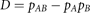where pA and pB denote the respective frequencies of alleles A and B.

Let Xl (respectively Xm) denote the dummy random variable equaling 1 when an individual carries the A allele at locus l (respectively B allele at locus m) and 0 otherwise. Then D=Cov(Xl, Xm). By definition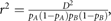thus it is equal to Cor2(Xl, Xm) as Var(Xl)=pA(1−pA) and Var(Xm)=pB(1−pB).

#### The case of sample structure

As in Ohta (1982), our aim is the decomposition of the r2 measure of linkage disequilibrium into a first part due to the sample structure and a second part clear of the structure effect. We prove that the part clear of the structure effect has two major properties. First, it is unbiased for unlinked loci, unlike the usual r2 measure of linkage disequilibrium. Second, it is the proportion factor of increase of the sample size necessary to achieve the same power when testing association at a SNP locus in linkage disequilibrium with the causal locus, under the linear Q model of association (Yu et al., 2006).

Let us illustrate the idea leading to the decomposition of the r2 measure of linkage disequilibrium by considering a simple situation. Assuming that gametes are sampled from two different populations, we denote by S the Bernoulli random variable that equals 1 when the gamete is sampled from the first population and 0 otherwise. If S is known, a natural statistical way of using this information, is to consider the conditional random variables XlS and XmS. We propose the square of their correlation as a novel measure of linkage disequilibrium and we denote this novel measure rS2.

When allele frequencies are different between populations, the r2 measure is not equal to zero for independent loci, as the ideal measure of linkage disequilibrium should be. Based on the following property of the conditional covariance,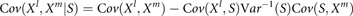it can be proved that rS2 is equal to 0 for independent loci and thus that it corrects the bias of the usual r2 measure (see APPENDIX A in Supplementary Information).

As defined, the novel measure allows a straightforward generalization to cases of samples composed of gametes coming from K different populations. The only changes needed are to set S=(S1, … , Sk, … , SK−1)T where Sk is the Bernoulli random variable that equals 1 when the gamete is sampled from the kth population and 0 otherwise, and to use the Cov and Var matrix extensions. The generalization to the case of admixed populations, which is modeled by a known mixture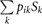on K ancestral groups for each individual i, raises no further problem.

We now derive the way to compute rS2 sample value. Having sampled N individuals, we consider the 2N derived gametes and the population structure matrix S of the gametes in K populations. The S matrix is composed of 2N rows and K−1 columns. It can be obtained from Bayesian model-based clustering methods, as in STRUCTURE (University of Chicago, IL, USA; Pritchard et al., 2000) and BAPS (Abo Akademi University, Turku, Finland; Corander et al., 2008) software applications, by removing the last column of the output structure matrix, or from dimension-reduction methods, such as principal component analysis (Patterson et al., 2006), non-metric multidimensional scaling (Zhu and Yu, 2009) or sparse factor analysis (Engelhardt and Stephens, 2010) by considering K−1 dimensions.

Let Xl, Xm be the 0–1 column vectors of the observed gametic alleles at loci l and m. We denote by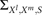the sample variance-covariance matrix of Xl, Xm and S. It consists in 3 × 3 blocks, namely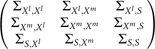Then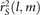the estimate of the rS2(l, m) measure is equal to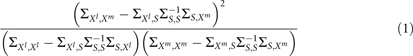The above equation is well defined if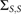is not singular. Otherwise, it can be proved with the help of linear algebraic theorems (Searle, 1971 (chapter 2, p. 37)) that the number of ancestral groups can be reduced without loss of information.

The measure on unphased genotypes (Weir, 1979; Rogers and Huff, 2009) is defined by the correlation between Xl and Xm where Xl and Xm are now multinomial random variables taking values 0, 1 or 2 according to the number of A alleles in the genome at locus l (respectively m). As the population structure matrix can be obtained for genotypes as well as for gametes, it is straightforward to extend the rS2 measure to phase-unknown genotypes.

We give here the main arguments to prove that the power of the detection test at a SNP locus linked to the causal polymorphism is equal to the power at the causal polymorphism if the sample size is increased by 1/rS2. The power of a t-test is an increasing function of its expectation, so we studied this expectation both at the causal locus and at a SNP locus in linkage disequilibrium. First, we show that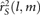is the factor that reduces the power when testing the association at the linked marker locus using the Q model and then we deal with two samples of different sizes. The complete proof is given in appendix (see APPENDIX B in Supplementary material).

#### The case of relatedness of sampled individuals

When the sample is composed of N related gametes, the sample correlation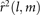is not a good estimate of the r2(l, m) measure of linkage disequilibrium as the independence assumption is violated. The same problem occurs for the sample value of the correlation conditionally to the structure, rS2(l, m). However, if the covariance between the pairs of gametes is known, then the observations can be decorrelated by premultiplying the data by V−1/2 if it exists, where V is a matrix built from the Vij covariances for all the (i, j) pairs of gametes. Assuming that V is invertible, we denote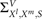the sample variance-covariance matrix of V−1/2[Xl, Xm, S] which is equal to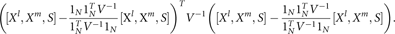We derive the estimate of the novel measure of linkage disequilibrium given the covariance between gametes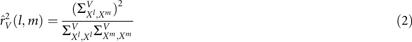and the estimate of the novel measure of linkage disequilibrium given both the covariance between gametes and the sample structurewhere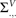denotes the blocks in the matrix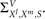When the V matrix is not invertible, we propose to replace V−1 in equations (2) and (3) by V, the generalized Moore–Penrose inverse which is always defined.

As in the case of sample structure, extension to genotypes of unknown phase is straightforward by replacing all matrices and data related to gametes by the corresponding genotypic information, with V becoming the covariance matrix between genotypes.

The kinship coefficient which represents the degree of genetic covariance among individuals is the genetic parameter that should be used in the V matrix. It has already been proposed in association mapping methods to remove the bias of the type I error of association tests due to different degrees of relatedness between individuals and it was shown to be adequate (Yu et al., 2006).

### Computer simulations

The aim was to show that these novel measures actually corrected the bias of the usual r2 estimate. Simulation process was conducted differently for the rS2 and the rV2 measures.

For the rS2 measure, each population was described by two parameters, pl, pm, the A (respectively B) allele frequency of the first (respectively the second) diallelic locus. The r2 measure of linkage disequilibrium was assumed to be the same in both populations. In each population, a N sample was simulated using a multinomial distribution M(1, pAB, pAb, paB, pab) where pAA, pAb, paB and pab were the frequencies of the four allelic combinations linked to the above parameters.

For consistency with our real data set on grapevine, simulations were conducted with a sample size of 100 genotypes per population. First, we studied three levels of linkage disequilibrium by setting r2 to 0.01, 0.25 and 0.5. The allelic frequencies were chosen in order to show a strong differentiation between the two populations either for both loci or for one locus only. Then, we explored the whole parameter space for r2, pl and pm (see Supplementary material).

For the rV2 measure, two series of simulations were carried out. The first one involved in simulating a sample composed of both independent and cloned genotypes. A total of 80 genotypes were obtained by randomly associating two gametes that were simulated as described above with the multinomial distribution. A single genotype, homozygous for the most common allele at both loci, was chosen and repeated 19 times in order to create a set of 20 cloned genotypes within the sample of 100 genotypes. The V matrix was the kinship matrix with 1 diagonal. The cloned genotypes were assigned a kinship coefficient equal to 1, whereas all other kinship coefficients were set to 0. We studied three levels of linkage disequilibrium (r2=0.05, 0.25, 0.4) and the allelic frequencies at both loci were chosen as either high (0.5) or medium (0.2).

The second series of simulations focused on a sample composed of full and half-sibs. A sample of 20 genotypes was simulated with a multinomial distribution. These 20 genotypes were used as the parents of a complete half-diallel of 190 F2 families with 200 members each. For a given genetic distance between the two loci, the genotype of an offspring was obtained from the genotypes of its parents using Mendelian laws. The allelic transmission from parents to sampled descendants was preserved across replicates in order to limit the randomness to the multinomial draw. Thus, the parent genotypes varied across the replicates and the genotypes of descendants were rebuilt given the allelic transmission and their parent genotypes. Two random samplings of the descendants were used: either 100 individuals were randomly sampled in the whole population (simple sampling) or 5 individuals were randomly sampled in 20 randomly drawn families (hierarchical sampling). The V matrix was the kinship matrix with 1 diagonal. The kinship coefficients between the descendants were set to 1/4, 1/8 or 0 depending on the number of parents they shared. We set r2 to 0.4, 0.25 and 0.05 and, based on a previous study on grapevine (Barnaud et al., 2010, Figure 1), we linked these values to 0.1, 1 and 5 cM, respectively.

For both scenarii, we had to limit the number of replicates compared with the structure simulations because computing the inverse of a matrix drastically slowed down the computation.

### Plant material

To illustrate the behavior of the novel linkage disequilibrium measures, we also analyzed a real stratified population obtained by mixing cultivated and wild grapevine accessions with different kinship levels. Respectively 92 and 91 plants were selected among the wild and cultivated accessions of the V. vinifera grapevine germplasm collection of the vassal INRA experimental station. The 91 cultivated accessions (CU sample) were selected in the ‘table east’ sub-population (table grape cultivars from Eastern Europe). This sub-population was one of the three ones obtained by stratification analysis with STRUCTURE software application, using 20 microsatellite markers spanning the 19 chromosomes on the 2276 distinct genotypes of the cultivated V. vinifera germplasm. The 92 wild accessions selected (WI sample) came mainly from Western Europe and most particularly from France.

### Molecular analysis

DNA was extracted from these 183 accessions and genotyped at 176 SNP markers using VeraCode technology (Illumina, San Diego, CA, USA). A total of 109 SNPs belonging to 50 different genes within a 2-Mb region were used to estimate linkage disequilibrium. In all, 67 additional SNPs originating from 67 different genes scattered on the whole genome were used together with the 20 scattered SSRs to measure relatedness between the 183 accessions. All SNPs were physically localized on the sequence of grapevine genome by blasting their flanking sequence on the 12 × reference grapevine genome sequence (Jaillon et al., 2007).

### Data analysis

Linkage disequilibrium analysis was performed for the 80 SNPs out of 109 with a minimum allele frequency >10%. We checked stratification between wild and cultivated grapevine accessions by performing a phylogenetic analysis based on the dissimilarity matrix estimated from the 87 markers scattered on the whole genome (see above). We obtained a clear classification into two genetic pools corresponding to wild and cultivated accessions (data not shown). The structure matrix was obtained from the same 87 scattered markers with STRUCTURE assuming no admixture. The kinship matrix was obtained with the same 87 scattered markers with ML-Relate (Montana state University, Bozeman, MT, USA), a software application that uses maximum likelihood to estimate relatedness between individuals (Milligan, 2003).

## Results

### Simulation study

#### Validation of the bias correction for structure

To validate the rS2 measure, simulations were conducted on a two-population structured sample.

Table 1 presents the mean (and its standard error) of the estimates of rS2 and r2, computed over 5000 replicates. The r2 measure was estimated both in the first population (100 genotypes) and in the whole sample (200 genotypes).

As expected, the higher the differentiation of the loci, the higher was the bias of the r2 estimate. In all cases, the rS2 measure corrected the bias of the r2 estimate in the whole sample. Depending on allele frequencies and linkage disequilibrium values, the r2 estimate either under or overestimated the r2 value but in all cases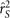was nearly unbiased. For the variability of the estimates, as shown by the standard error, the trends were opposite. The difference invariance between the one and two-differentiated locus situations decreased with increasing linkage disequilibrium whereas the opposite was observed for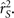However, the trends for the variability of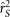and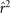in the first population, were the same, suggesting that this is the usual trend of a correct estimate of linkage disequilibrium based on correlation across gametes.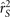showed less unbiasedness and a smaller variance than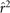in the first population because the sample size was twice as large.

The rS2 estimate was almost unbiased over the whole parameter space, as the absolute difference between its mean over the 500 replicates and the r2 value was always <0.03 (see Supplementary material).

#### Validation of the bias correction for relatedness

To validate the rV2 measure, two scenarii were simulated, a clone scenario and a half-diallel design. Tables 2 and 3 present the mean (and its standard error) of the estimates of rV2 and r2, over 500 replicates. In the clone scenario, the large overestimation of linkage disequilibrium when using the r2 measure was expected since the cloned genotype was the most common haplotype. In the half diallel design, as parents could have different haplotypes and they all produced the same numbers of offspring, the impact on the bias of r2 estimate was not predictable. This bias was largely positive for medium allele frequencies whereas it was positive but nearly null for common alleles.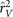was always less biased than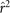. Bias correction was nearly perfect for common alleles but bias was only partially removed for medium allelic frequencies in the worst situation of simple population sampling with low r2. Variability was very close between r2 and rV2 estimates. It was substantially larger in the half-diallel design than in the clone scenario, most probably because the effective population size was much lower in the half-diallel (only 20 parents) than in the clone scenario (80).

### Grapevine data

r2 and rVS2 estimates are presented in Figure 1 as a function of the physical distance between loci. With the r2 measure, long-range linkage disequilibrium was questionable and could be due to either the structure of the sample and/or the high mean level of relatedness (0.32) within the wild sample as compared with the cultivated one (0.10). With the rVS2 measure, lower values overall were obtained, as well as an expected exponential decline of linkage disequilibrium with distance, which clearly demonstrated the efficiency of our novel measure in correcting bias.

Figure 2 shows the difference between the estimates of r2 and rVS2, plotted as a function of the distance between loci. The positive bias was removed all along the chromosome segment. However, for some close loci, rVS2 estimate was larger than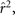leading to a negative removed bias.

## Discussion

We proposed two novel measures of linkage disequilibrium that correct for the bias of the usual r2 measure due to the structure of the sample or the relatedness of the genotyped individuals. These two measures can be combined in order to correct for both sources of bias and can be computed either on phased or unphased genotypes. Estimation of these novel measures of linkage disequilibrium was implemented in a R package (LDcorSV) and integrated in the SNiPlay pipeline, which is freely available at http://sniplay.cirad.fr/cgi-bin/home.cgi. These measures are based on formulae involving the covariance between gametes or diploid genotypes. They make full use of the added information given by the population structure or kinship matrices.

We proved, in the case of a two-population structured sample, that the estimate of the measure corrected for the structure of the sample, rS2, is unbiased for unlinked loci. We showed by simulation of a two-population structured sample that the bias of the usual estimate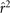increased with the differentiation of loci and with decreasing linkage disequilibrium, as already shown by Ohta (1982). This bias was always removed by the use of the rS2 estimate. Our simulations were limited to two populations having different levels of linkage disequilibrium only because of different allele frequencies. Both populations thus have similar r2 values and an unbiased estimate of r2 can be clearly defined as an estimate with expectation r2. On the contrary, in the case where the two populations would have both different r2 values and different allele frequencies, it is not clear what should be the correct measure of linkage disequilibrium, and consequently what would be the bias of the usual r2 estimate. If two populations diverged a long time ago and underwent very different evolutions, it may be wrong to assume that they differ only in allele frequencies. However, for geographically close populations, for recently admixed populations, or for genotypes pooled to form a panel for association studies, assuming the r2 value is the same is not unsoundness. Moreover, if we assume equal effective population sizes and panmixia in each population, the approximation of the expectation of r2 along the evolution process depends on Nc, where N is the effective population size and c the per-generation rate of recombination (Ohta and Kimura, 1971). Therefore, r2 may be linked to the recombination rate, which is expected to be similar in two close populations.

Another extreme clone scenario could be useful to show that the r2 measure may also underestimate the true linkage disequilibrium value, in which case the rV2 estimate would be much larger than the usual r2 estimate. Let nAB (respectively nab) denote the number of haplotypes carrying A allele at the first locus and B allele at the second locus (a and b alleles, respectively). If there were only one haplotype carrying Ab and one haplotype carrying aB, the r2 estimated value of the sample would be large, nearly equal to 1 if nAB and nab were large. However, if the single Ab haplotype and the single aB haplotype were both cloned, then the r2 estimated value of this new sample would decrease to zero with an increasing number of clones. Unlike the r2 estimate, the rV2 estimate would remain equal to the r2 estimate of the original sample and would thus be nearly unbiased for a large sample size. By simulating a diallel design of full-sibs, which is less extreme and thus more likely to reflect real situations, we showed that the usual measure was always more biased than the novel one. However, we observed a remaining bias that was probably due to the small effective size of our sample as observed by Yan et al. (2009) with a real maize data set when the sample size was <50.

Analyses of the real data set revealed a number of close loci with a linkage disequilibrium underestimated by the usual measure. This negative bias appeared even when we performed exclusively the structure correction (Supplementary Figure S1 in Supplementary material), although it was never found in the simulation study. The reason is probably that we performed simulations with exactly the same r2 value in both populations, as our goal was to estimate the part of linkage disequilibrium linked to the recombination rate within populations in panmixia. In our real data set, these pairs of close loci showed a significant difference of r2 estimates between populations. However, among these pairs, those with the highest differences between r2 and rS2, had low allelic frequency for one locus within at least one population (Supplementary Figure S2 in Supplementary material). Given the small sample size within each population (<100 accessions), one must proceed with caution when drawing conclusions but this suggests that those loci had probably undergone selection.

Recently, Zaykin et al. (2008) proposed an extension of the r2 measure to handle multiallelic loci. Their T2 statistic, which is the sum of the r2 measures over all pairs of alleles multiplied by a constant term, was shown to be efficient for testing for independence between loci in phase-known genotypes. The transformations using population structure and kinship matrices could be applied to the T2 statistics to correct for bias effects.

Kinship matrices are rarely known and there are several methods and software applications for obtaining an estimated kinship matrix based on molecular marker data (Loiselle et al., 1995; Lynch and Ritland, 1999; Milligan, 2003). However, they all have the limited goal of estimating pairwise kinship coefficients, none of them estimates a whole kinship matrix. The estimates of pairwise kinship coefficients of the whole sample, even when all of them are constrained to be positive or null, do not provide a valid matrix for the rV2 measure (that is, a positive semi-definite matrix). The simplest method of building a positive semi-definite matrix from a non-positive one, is to perform a single value decomposition and set all negative eigenvalues to 0. We used this method to analyze our real data set. However, as highlighted by Saïdou et al. (2009), this projection method does not necessarily lead to a valid kinship matrix (that is, a matrix with elements >0 and <1). Which is the best method when computing the rV2 measure, either to use a valid kinship matrix or simply a valid variance-covariance matrix, remains an open question. In the association mapping context with a mixed model approach, Kang et al. (2008) compared three different variance-covariance matrices of the random genotype background effect: a SPAGeDi-based kinship estimate, a genotype similarity estimate and a phylogeny control estimate. Based on both the BIC criterion, which measures the goodness of fit of the model, and the P-value distribution, which shows the correction needed for inflated false positive tests, the three estimates gave comparable results. For linkage disequilibrium estimation, we do not have a clear criterion, such as the BIC or the P-value distribution, that could help us to compare different variance-covariance matrices on real data sets. However, we may rely on Kang et al. (2008) comparisons to suggest that comparable results would probably be obtained.

We proved that the sample size has to be increased by 1/rS2 to achieve the same power at a SNP locus in linkage disequilibrium with a causal locus when using the association test corrected by the sample structure (the Q model of Yu et al., 2006). If V is the variance-covariance matrix of the K+Q model (Yu et al., 2006), then the rVS2 measure can be proved to be the proportional factor of the association test power. The proof follows the same steps as for the rS measure, after premultiplying the K+Q model equation by V−1/2. The variance-covariance matrix of this mixed linear model is generally unknown, as it depends on the heritability of the observed trait. However, the rVS2 measure could be computed for a range of heritabilities to help to select the marker density, or could be estimated after the association tests in order to assess the precision around the detected SNPs.